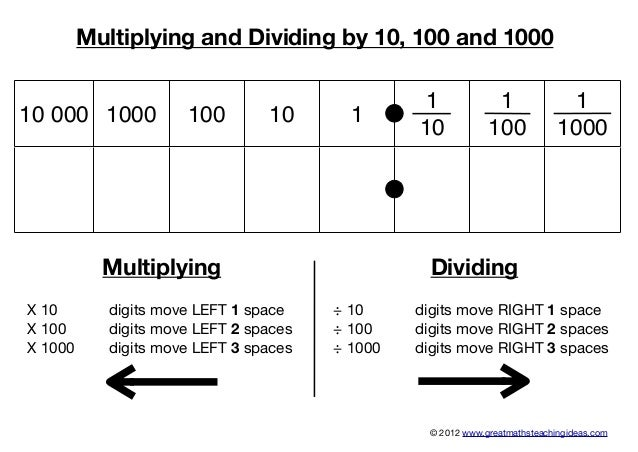In Year 2 children are required to learn their 2, 3, 4, 5 & 10 times table multiplication facts, applying these to use division facts. We call these 'inverse' facts.

Tables will be tested fortnightly. Further information will follow.

Tools to help us!

We will be looking at different tools to help us practise our multiplication and division facts.

A popular game to play is 'Hit the Button'.There are many helpful songs and videos available online. In class we often use 'Jack Haartman'.Methods we will be using:

Multiplying & Dividing by 10

Practise using your multiplication facts in different ways.

Switch -it:  2 x 10 = 20       10 x 2 = 20

Inverse: 20 divided by 10 = 2        20 divided by 2 = 10

Practise these strategies using your 10 times table facts.Why not extend to multiplying and dividing by 100 as an extra challenge!

(These will not be tested, but are great fun to practise)

2 x 10 = 20           2 x 100 = 200

20 divided by 10 = 2    200 divided by 100 = 2A great game to play to practise multiplying and dividing by 10 and 100 is:Mulitplying & Dividing by 5

Practise using your multiplication facts in different ways as we do in class:

Switch -it:  2 x 5 = 10          5 x 2 = 10

Inverse: 10 divided by 5 = 2        10 divided by 2 = 5

Practise these strategies using your 5 times table facts.Why not try using the darboard!Doubling & Halving: Linking our 2s and 4s

Continue to practise both of these tables, using doubling and halving strategies.

E.g 2 x 2 = 4

4 x 4 = 8Two Times TableThree times table